# Data Representation COE 205 Computer Organization and Assembly

• Slides: 33Data Representation COE 205 Computer Organization and Assembly Language Dr. Aiman El-Maleh College of Computer Sciences and Engineering King Fahd University of Petroleum and Minerals [Adapted from slides of Dr. Kip Irvine: Assembly Language for Intel-Based Computers]Outline v Introduction v Numbering Systems v Binary & Hexadecimal Numbers v Base Conversions v Integer Storage Sizes v Binary and Hexadecimal Addition v Signed Integers and 2's Complement Notation v Binary and Hexadecimal subtraction v Carry and Overflow v Character Storage Basic Concepts COE 205 – Computer Organization and Assembly Language – KFUPM slide 2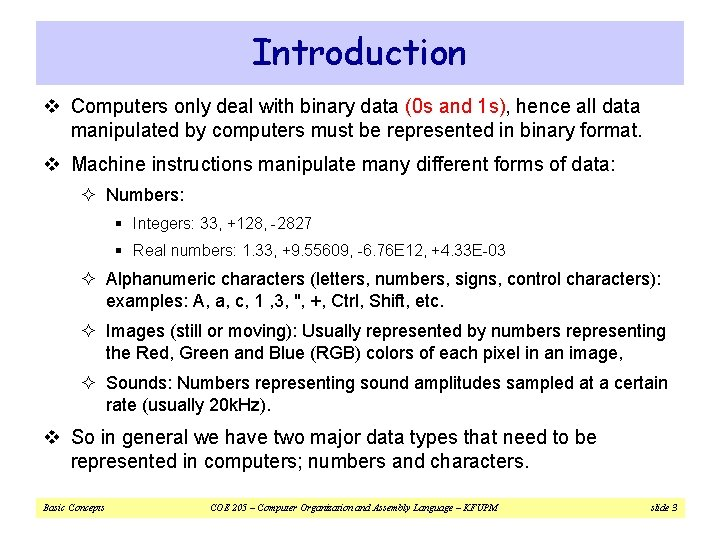Introduction v Computers only deal with binary data (0 s and 1 s), hence all data manipulated by computers must be represented in binary format. v Machine instructions manipulate many different forms of data: ² Numbers: § Integers: 33, +128, -2827 § Real numbers: 1. 33, +9. 55609, -6. 76 E 12, +4. 33 E-03 ² Alphanumeric characters (letters, numbers, signs, control characters): examples: A, a, c, 1 , 3, ", +, Ctrl, Shift, etc. ² Images (still or moving): Usually represented by numbers representing the Red, Green and Blue (RGB) colors of each pixel in an image, ² Sounds: Numbers representing sound amplitudes sampled at a certain rate (usually 20 k. Hz). v So in general we have two major data types that need to be represented in computers; numbers and characters. Basic Concepts COE 205 – Computer Organization and Assembly Language – KFUPM slide 3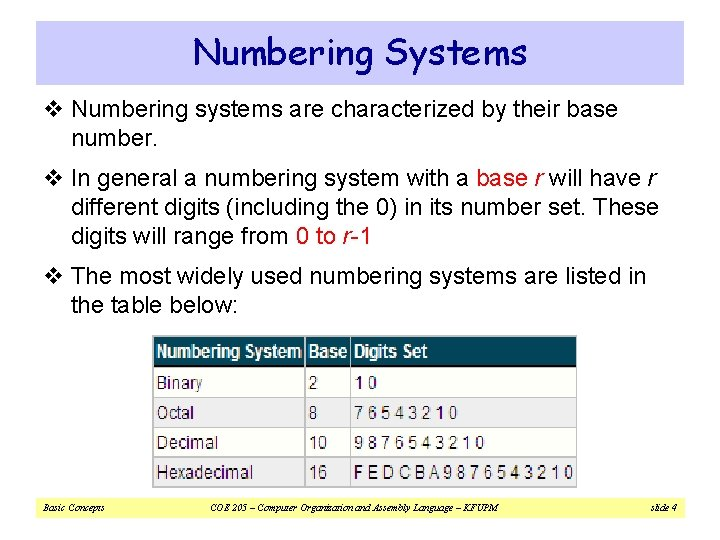Numbering Systems v Numbering systems are characterized by their base number. v In general a numbering system with a base r will have r different digits (including the 0) in its number set. These digits will range from 0 to r-1 v The most widely used numbering systems are listed in the table below: Basic Concepts COE 205 – Computer Organization and Assembly Language – KFUPM slide 4Binary Numbers v Each digit (bit) is either 1 or 0 v Each bit represents a power of 2 v Every binary number is a sum of powers of 2 Basic Concepts COE 205 – Computer Organization and Assembly Language – KFUPM slide 5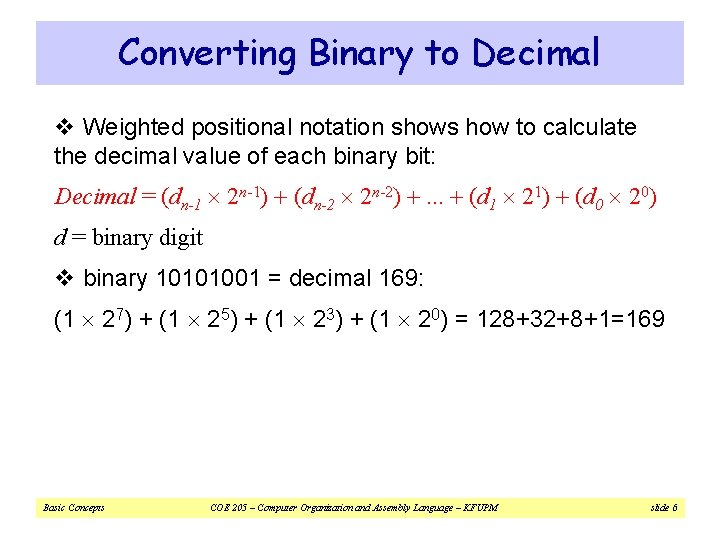Converting Binary to Decimal v Weighted positional notation shows how to calculate the decimal value of each binary bit: Decimal = (dn-1 2 n-1) + (dn-2 2 n-2) +. . . + (d 1 21) + (d 0 20) d = binary digit v binary 10101001 = decimal 169: (1 27) + (1 25) + (1 23) + (1 20) = 128+32+8+1=169 Basic Concepts COE 205 – Computer Organization and Assembly Language – KFUPM slide 6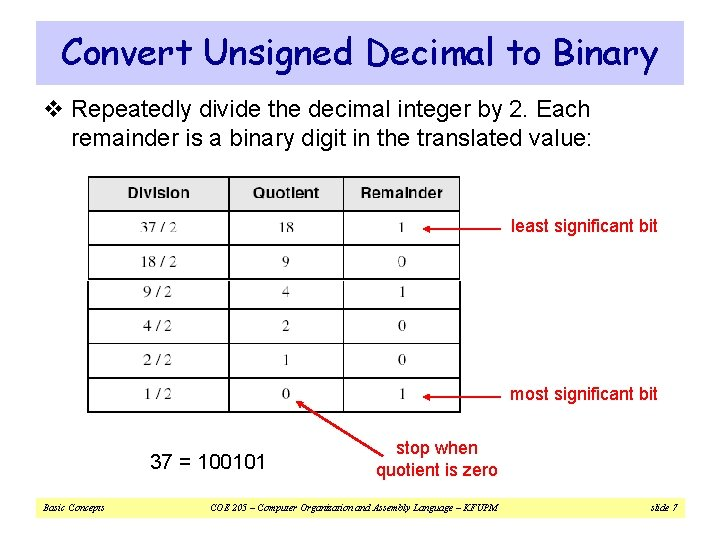Convert Unsigned Decimal to Binary v Repeatedly divide the decimal integer by 2. Each remainder is a binary digit in the translated value: least significant bit most significant bit 37 = 100101 Basic Concepts stop when quotient is zero COE 205 – Computer Organization and Assembly Language – KFUPM slide 7Another Procedure for Converting from Decimal to Binary v Start with a binary representation of all 0’s v Determine the highest possible power of two that is less or equal to the number. v Put a 1 in the bit position corresponding to the highest power of two found above. v Subtract the highest power of two found above from the number. v Repeat the process for the remaining number Basic Concepts COE 205 – Computer Organization and Assembly Language – KFUPM slide 8Another Procedure for Converting from Decimal to Binary v Example: Converting 76 d to Binary ² The highest power of 2 less or equal to 76 is 64, hence the seventh (MSB) bit is 1 ² Subtracting 64 from 76 we get 12. ² The highest power of 2 less or equal to 12 is 8, hence the fourth bit position is 1 ² We subtract 8 from 12 and get 4. ² The highest power of 2 less or equal to 4 is 4, hence third bit position is 1 ² Subtracting 4 from 4 yield a zero, hence all the left bits are set to 0 to yield the final answer Basic Concepts COE 205 – Computer Organization and Assembly Language – KFUPM slide 9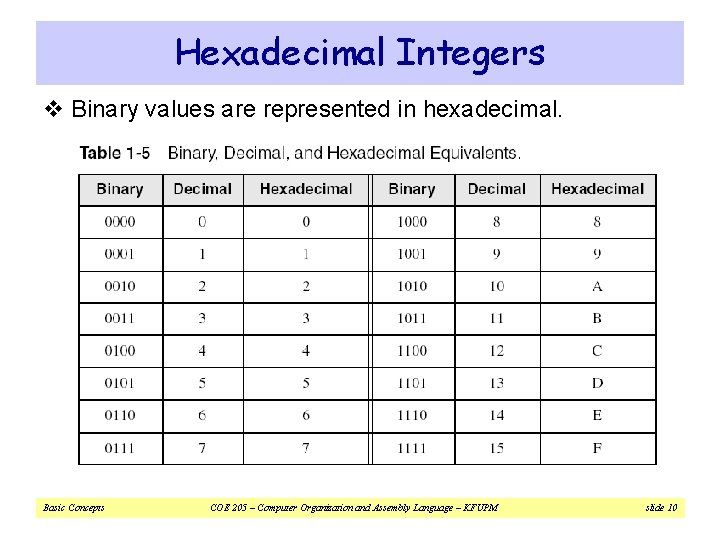Hexadecimal Integers v Binary values are represented in hexadecimal. Basic Concepts COE 205 – Computer Organization and Assembly Language – KFUPM slide 10Converting Binary to Hexadecimal v Each hexadecimal digit corresponds to 4 binary bits. v Example: Translate the binary integer 000101101010011110010100 to hexadecimal Basic Concepts COE 205 – Computer Organization and Assembly Language – KFUPM slide 11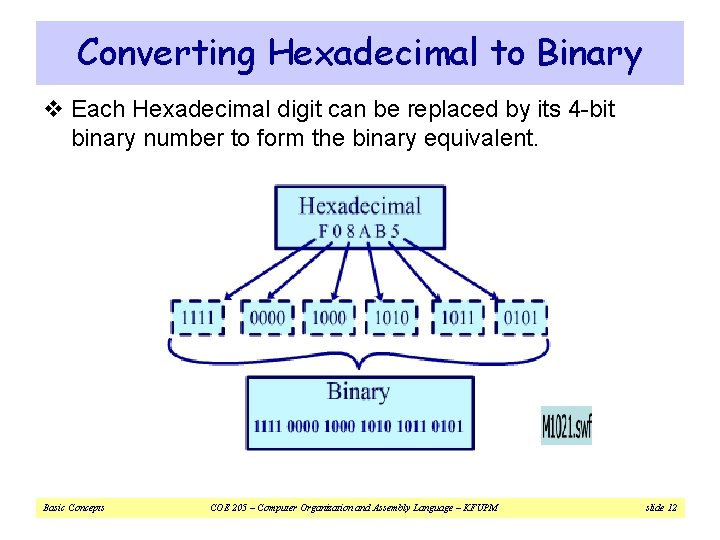Converting Hexadecimal to Binary v Each Hexadecimal digit can be replaced by its 4 -bit binary number to form the binary equivalent. Basic Concepts COE 205 – Computer Organization and Assembly Language – KFUPM slide 12Converting Hexadecimal to Decimal v Multiply each digit by its corresponding power of 16: Decimal = (d 3 163) + (d 2 162) + (d 1 161) + (d 0 160) d = hexadecimal digit v Examples: ² Hex 1234 = (1 163) + (2 162) + (3 161) + (4 160) = Decimal 4, 660 ² Hex 3 BA 4 = (3 163) + (11 * 162) + (10 161) + (4 160) = Decimal 15, 268 Basic Concepts COE 205 – Computer Organization and Assembly Language – KFUPM slide 13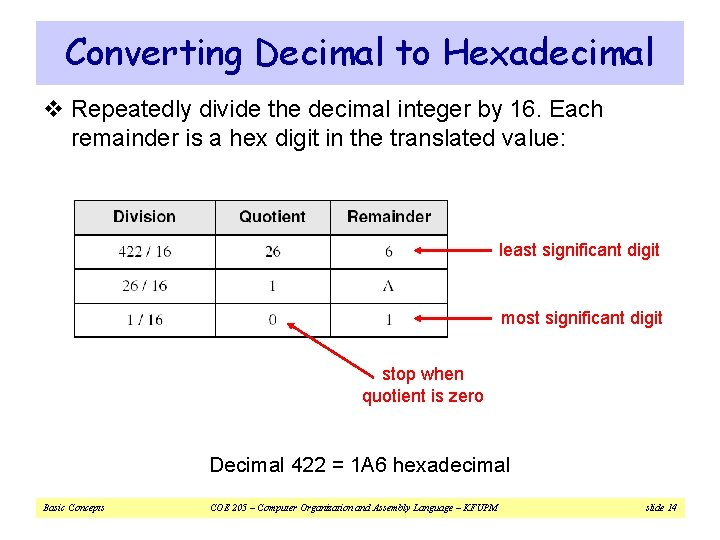Converting Decimal to Hexadecimal v Repeatedly divide the decimal integer by 16. Each remainder is a hex digit in the translated value: least significant digit most significant digit stop when quotient is zero Decimal 422 = 1 A 6 hexadecimal Basic Concepts COE 205 – Computer Organization and Assembly Language – KFUPM slide 14Integer Storage Sizes Standard sizes: What is the largest unsigned integer that may be stored in 20 bits? Basic Concepts COE 205 – Computer Organization and Assembly Language – KFUPM slide 15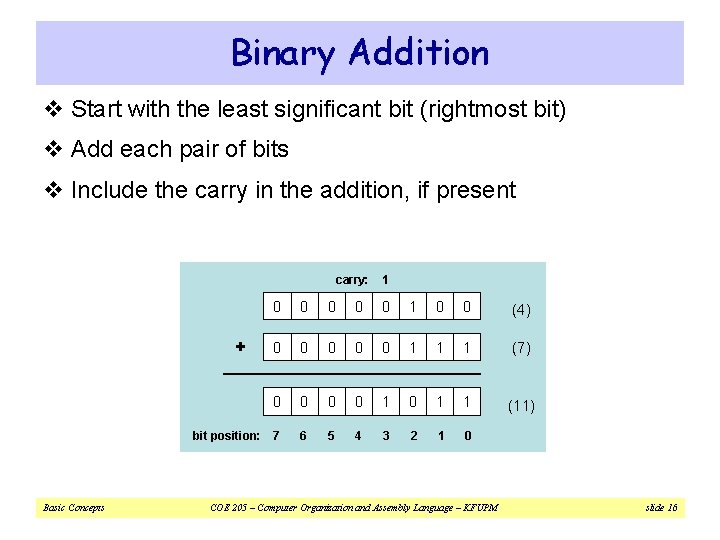Binary Addition v Start with the least significant bit (rightmost bit) v Add each pair of bits v Include the carry in the addition, if present carry: 0 0 0 1 0 0 (4) 0 0 0 1 1 1 (7) 0 0 1 0 1 1 (11) bit position: 7 6 5 4 3 2 1 0 + Basic Concepts 1 COE 205 – Computer Organization and Assembly Language – KFUPM slide 16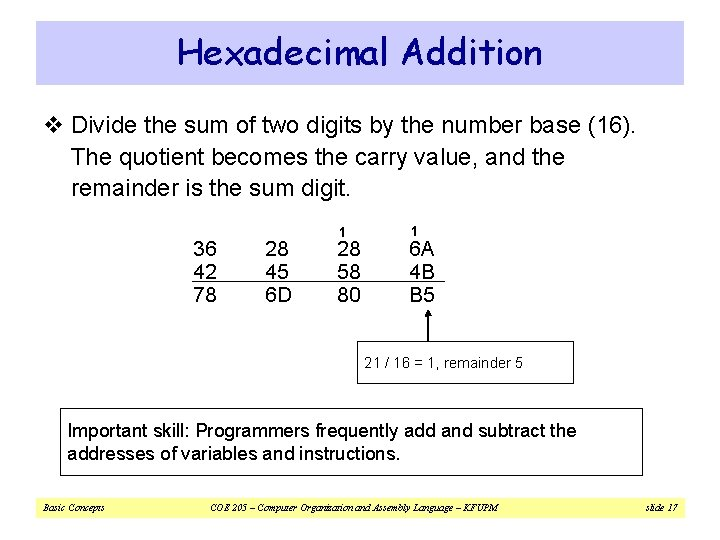Hexadecimal Addition v Divide the sum of two digits by the number base (16). The quotient becomes the carry value, and the remainder is the sum digit. 36 42 78 28 45 6 D 1 28 58 80 1 6 A 4 B B 5 21 / 16 = 1, remainder 5 Important skill: Programmers frequently add and subtract the addresses of variables and instructions. Basic Concepts COE 205 – Computer Organization and Assembly Language – KFUPM slide 17Signed Integers v Several ways to represent a signed number ² Sign-Magnitude ² 1's complement ² 2's complement v Divide the range of values into 2 equal parts ² First part corresponds to the positive numbers (≥ 0) ² Second part correspond to the negative numbers (< 0) v Focus will be on the 2's complement representation ² Has many advantages over other representations ² Used widely in processors to represent signed integers Basic Concepts COE 205 – Computer Organization and Assembly Language – KFUPM slide 18Two's Complement Representation v Positive numbers ² Signed value = Unsigned value v Negative numbers ² Signed value = Unsigned value - 2 n ² n = number of bits v Negative weight for MSB ² Another way to obtain the signed value is to assign a negative weight to most-significant bit 1 0 -128 64 1 1 0 0 32 16 8 4 2 1 = -128 + 32 + 16 + 4 = -76 Basic Concepts 8 -bit Binary Unsigned value Signed value 0000 0 0 00000001 1 +1 00000010 2 +2 . . 01111110 126 +126 01111111 127 +127 10000000 128 -128 10000001 129 -127 . . 11111110 254 -2 1111 255 -1 COE 205 – Computer Organization and Assembly Language – KFUPM slide 19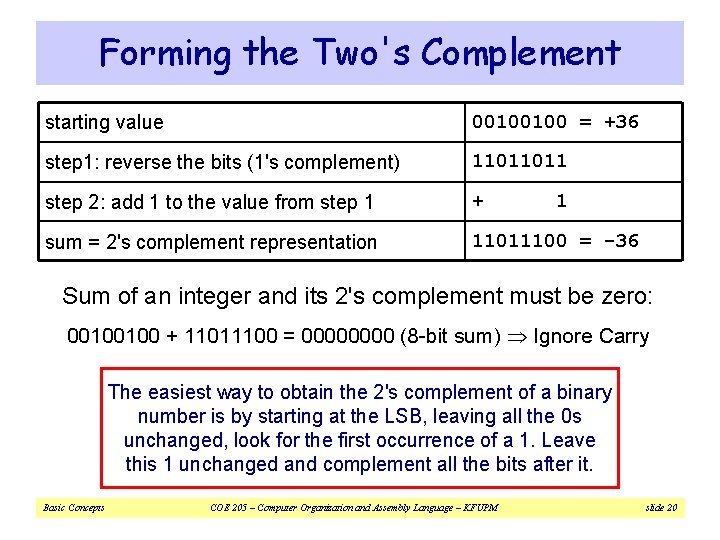Forming the Two's Complement starting value 00100100 = +36 step 1: reverse the bits (1's complement) 11011011 step 2: add 1 to the value from step 1 + sum = 2's complement representation 11011100 = -36 1 Sum of an integer and its 2's complement must be zero: 00100100 + 11011100 = 0000 (8 -bit sum) Ignore Carry The easiest way to obtain the 2's complement of a binary number is by starting at the LSB, leaving all the 0 s unchanged, look for the first occurrence of a 1. Leave this 1 unchanged and complement all the bits after it. Basic Concepts COE 205 – Computer Organization and Assembly Language – KFUPM slide 20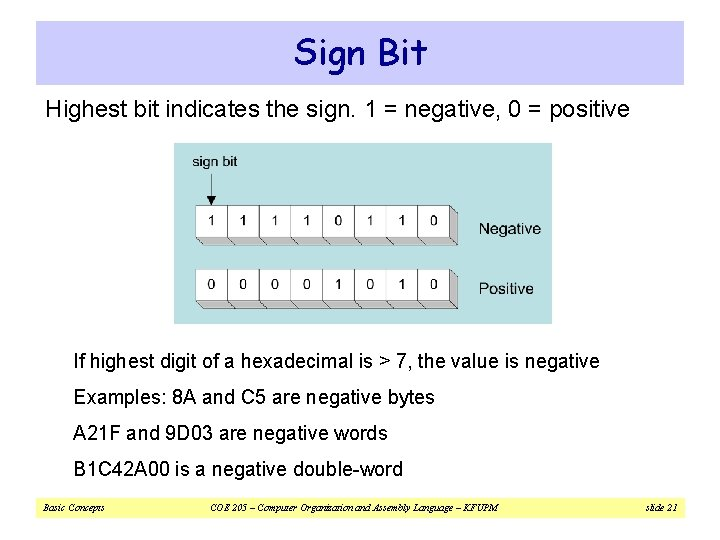Sign Bit Highest bit indicates the sign. 1 = negative, 0 = positive If highest digit of a hexadecimal is > 7, the value is negative Examples: 8 A and C 5 are negative bytes A 21 F and 9 D 03 are negative words B 1 C 42 A 00 is a negative double-word Basic Concepts COE 205 – Computer Organization and Assembly Language – KFUPM slide 21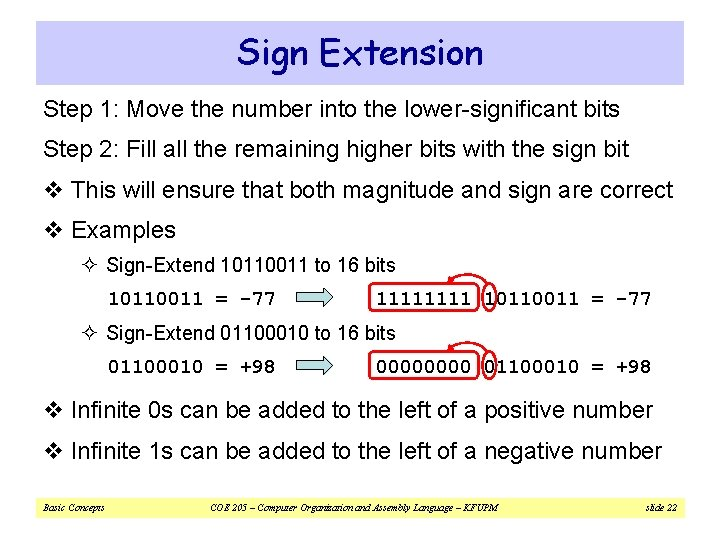Sign Extension Step 1: Move the number into the lower-significant bits Step 2: Fill all the remaining higher bits with the sign bit v This will ensure that both magnitude and sign are correct v Examples ² Sign-Extend 10110011 to 16 bits 10110011 = -77 1111 10110011 = -77 ² Sign-Extend 01100010 to 16 bits 01100010 = +98 0000 01100010 = +98 v Infinite 0 s can be added to the left of a positive number v Infinite 1 s can be added to the left of a negative number Basic Concepts COE 205 – Computer Organization and Assembly Language – KFUPM slide 22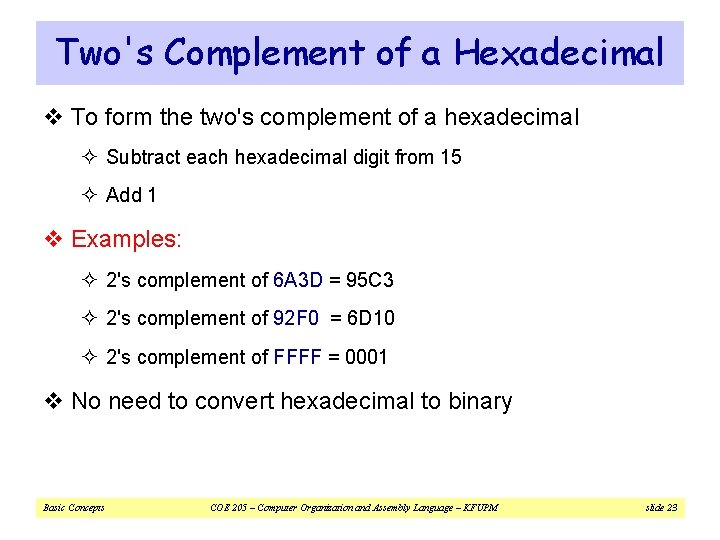Two's Complement of a Hexadecimal v To form the two's complement of a hexadecimal ² Subtract each hexadecimal digit from 15 ² Add 1 v Examples: ² 2's complement of 6 A 3 D = 95 C 3 ² 2's complement of 92 F 0 = 6 D 10 ² 2's complement of FFFF = 0001 v No need to convert hexadecimal to binary Basic Concepts COE 205 – Computer Organization and Assembly Language – KFUPM slide 23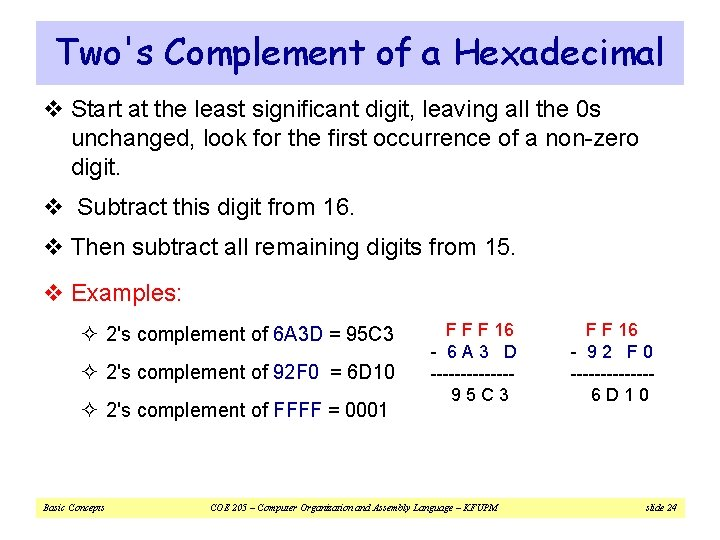Two's Complement of a Hexadecimal v Start at the least significant digit, leaving all the 0 s unchanged, look for the first occurrence of a non-zero digit. v Subtract this digit from 16. v Then subtract all remaining digits from 15. v Examples: ² 2's complement of 6 A 3 D = 95 C 3 ² 2's complement of 92 F 0 = 6 D 10 ² 2's complement of FFFF = 0001 Basic Concepts F F F 16 - 6 A 3 D -------95 C 3 COE 205 – Computer Organization and Assembly Language – KFUPM F F 16 - 92 F 0 -------6 D 10 slide 24Binary Subtraction v When subtracting A – B, convert B to its 2's complement v Add A to (–B) – 00001100 0000001010 + 00001100 11111110 (2's complement) 00001010 (same result) v Carry is ignored, because ² Negative number is sign-extended with 1's ² You can imagine infinite 1's to the left of a negative number ² Adding the carry to the extended 1's produces extended zeros Practice: Subtract 00100101 from 01101001. Basic Concepts COE 205 – Computer Organization and Assembly Language – KFUPM slide 25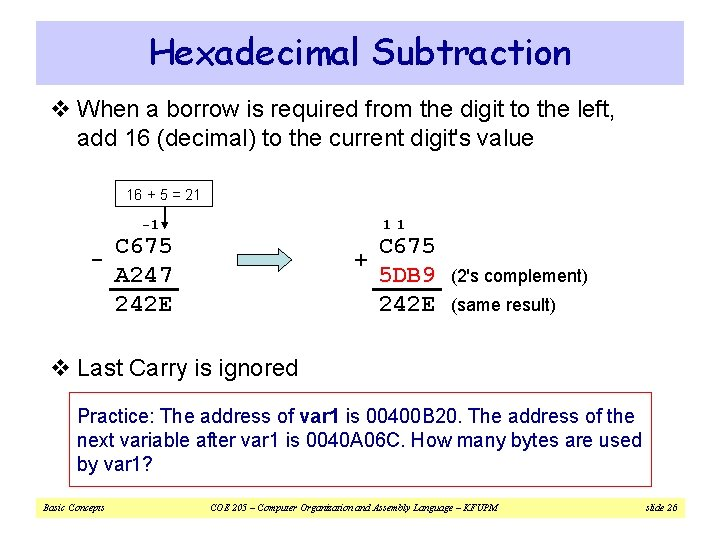Hexadecimal Subtraction v When a borrow is required from the digit to the left, add 16 (decimal) to the current digit's value 16 + 5 = 21 -1 - 11 C 675 A 247 242 E + C 675 5 DB 9 242 E (2's complement) (same result) v Last Carry is ignored Practice: The address of var 1 is 00400 B 20. The address of the next variable after var 1 is 0040 A 06 C. How many bytes are used by var 1? Basic Concepts COE 205 – Computer Organization and Assembly Language – KFUPM slide 26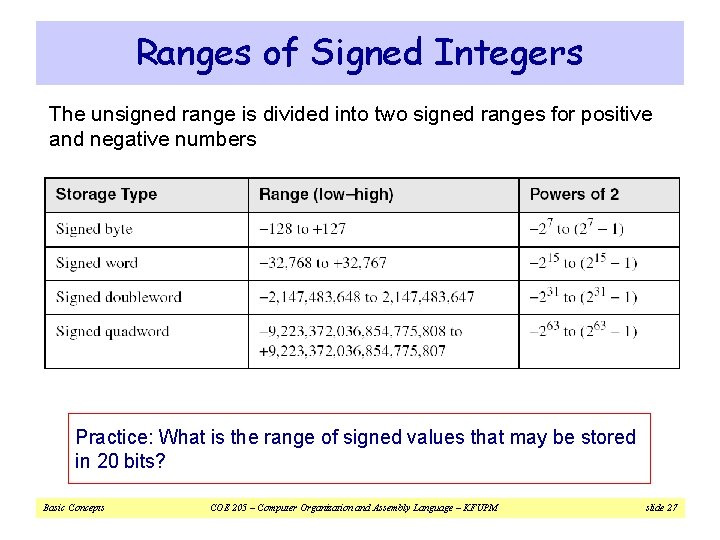Ranges of Signed Integers The unsigned range is divided into two signed ranges for positive and negative numbers Practice: What is the range of signed values that may be stored in 20 bits? Basic Concepts COE 205 – Computer Organization and Assembly Language – KFUPM slide 27Carry and Overflow v Carry is important when … ² Adding or subtracting unsigned integers ² Indicates that the unsigned sum is out of range ² Either < 0 or > maximum unsigned n-bit value v Overflow is important when … ² Adding or subtracting signed integers ² Indicates that the signed sum is out of range v Overflow occurs when ² Adding two positive numbers and the sum is negative ² Adding two negative numbers and the sum is positive ² Can happen because of the fixed number of sum bits Basic Concepts COE 205 – Computer Organization and Assembly Language – KFUPM slide 28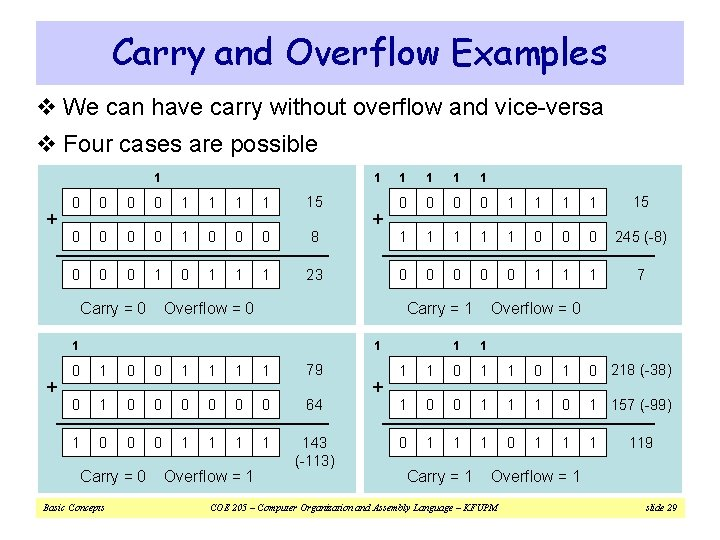Carry and Overflow Examples v We can have carry without overflow and vice-versa v Four cases are possible 1 + 1 0 0 1 1 15 0 0 1 0 0 0 8 0 0 0 1 1 1 23 Carry = 0 Overflow = 0 1 1 1 0 0 1 1 15 1 1 1 0 0 0 245 (-8) 0 0 0 1 1 1 7 Carry = 1 1 + + 1 1 0 0 1 1 79 0 1 0 0 0 64 1 0 0 0 1 1 143 (-113) Carry = 0 Basic Concepts Overflow = 1 + Overflow = 0 1 1 0 218 (-38) 1 0 0 1 1 1 0 1 157 (-99) 0 1 1 1 Carry = 1 119 Overflow = 1 COE 205 – Computer Organization and Assembly Language – KFUPM slide 29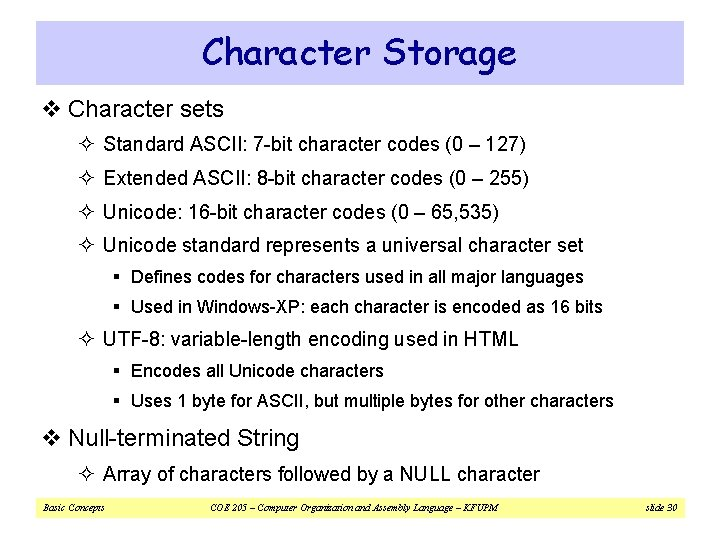Character Storage v Character sets ² Standard ASCII: 7 -bit character codes (0 – 127) ² Extended ASCII: 8 -bit character codes (0 – 255) ² Unicode: 16 -bit character codes (0 – 65, 535) ² Unicode standard represents a universal character set § Defines codes for characters used in all major languages § Used in Windows-XP: each character is encoded as 16 bits ² UTF-8: variable-length encoding used in HTML § Encodes all Unicode characters § Uses 1 byte for ASCII, but multiple bytes for other characters v Null-terminated String ² Array of characters followed by a NULL character Basic Concepts COE 205 – Computer Organization and Assembly Language – KFUPM slide 30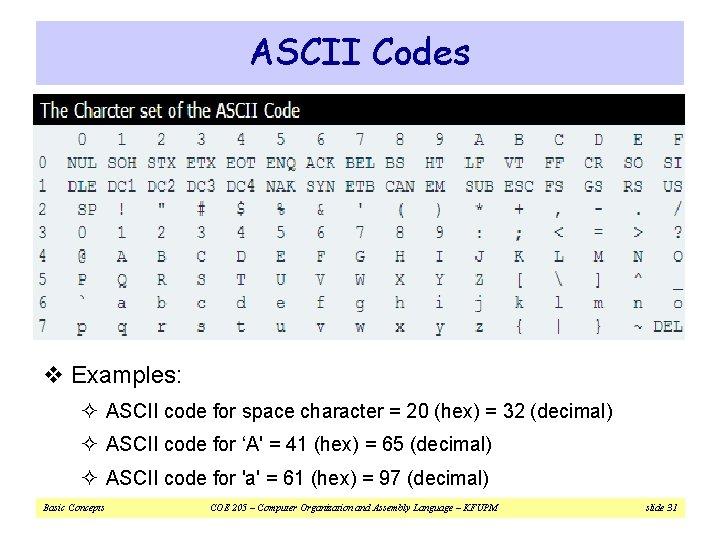ASCII Codes v Examples: ² ASCII code for space character = 20 (hex) = 32 (decimal) ² ASCII code for ‘A' = 41 (hex) = 65 (decimal) ² ASCII code for 'a' = 61 (hex) = 97 (decimal) Basic Concepts COE 205 – Computer Organization and Assembly Language – KFUPM slide 31Control Characters v The first 32 characters of ASCII table are used for control v Control character codes = 00 to 1 F (hex) v Examples of Control Characters ² Character 0 is the NULL character used to terminate a string ² Character 9 is the Horizontal Tab (HT) character ² Character 0 A (hex) = 10 (decimal) is the Line Feed (LF) ² Character 0 D (hex) = 13 (decimal) is the Carriage Return (CR) ² The LF and CR characters are used together § They advance the cursor to the beginning of next line v One control character appears at end of ASCII table ² Character 7 F (hex) is the Delete (DEL) character Basic Concepts COE 205 – Computer Organization and Assembly Language – KFUPM slide 32Parity Bit v Data errors can occur during data transmission or storage/retrieval. v The 8 th bit in the ASCII code is used for error checking. v This bit is usually referred to as the parity bit. v There are two ways for error checking: ² Even Parity: Where the 8 th bit is set such that the total number of 1 s in the 8 -bit code word is even. ² Odd Parity: The 8 th bit is set such that the total number of 1 s in the 8 -bit code word is odd. Basic Concepts COE 205 – Computer Organization and Assembly Language – KFUPM slide 33